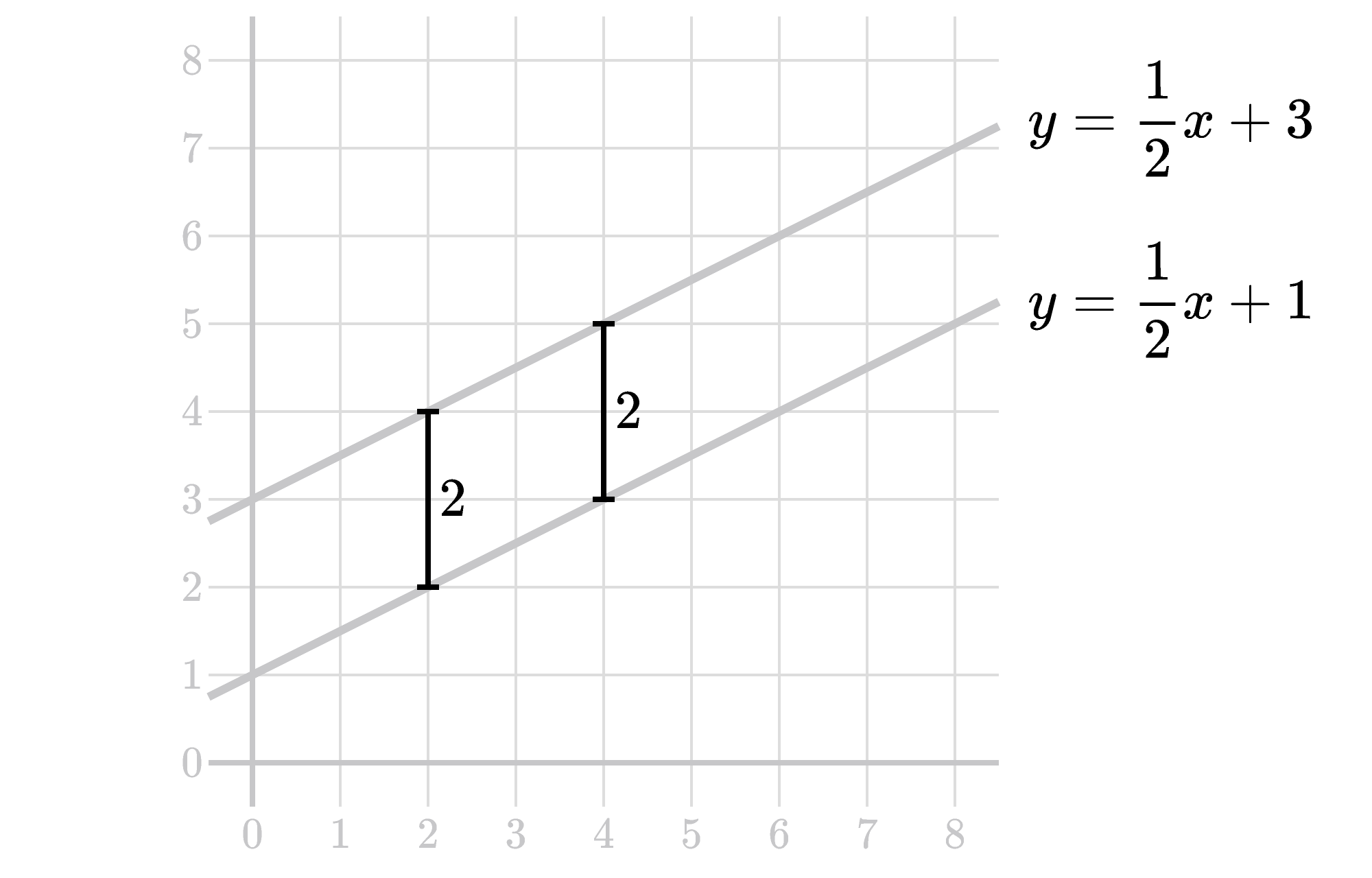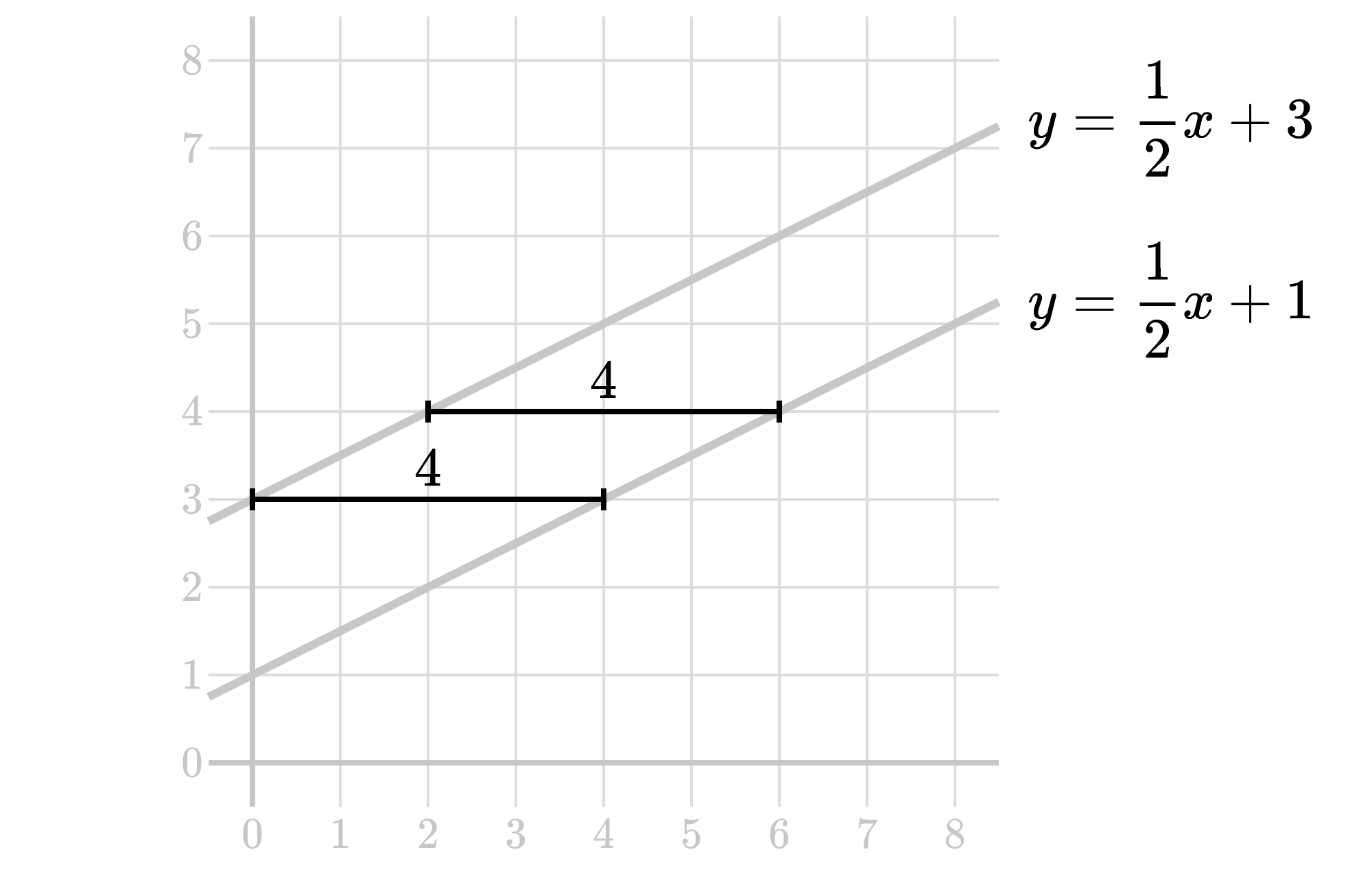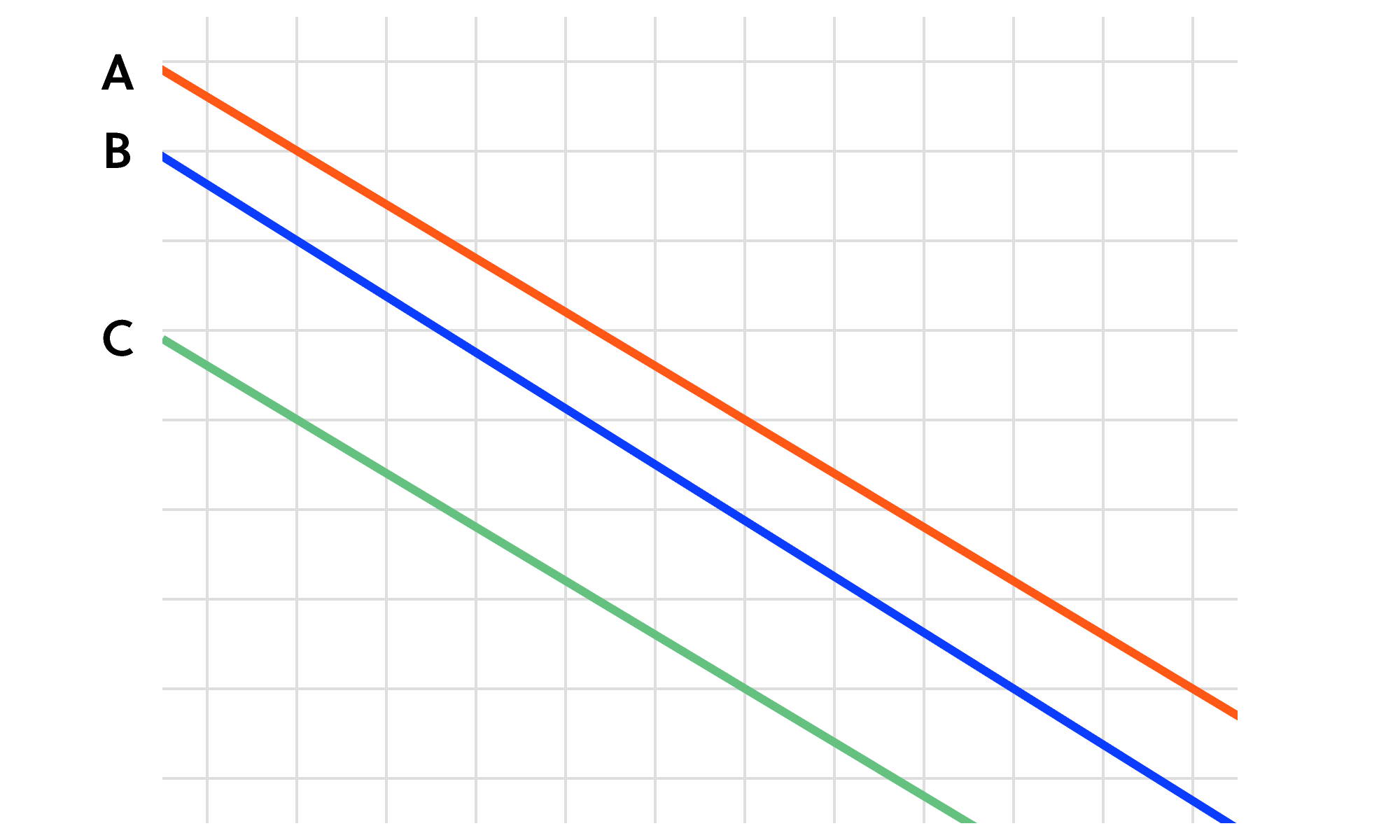Back

## Parallel or Not?

How can we tell if two lines are parallel? Lines go on forever, so it isn’t possible to examine an entire line to be sure that it doesn’t intersect the other. But there are more practical ways to tell, and the best method depends on how the lines are presented.

When lines are given as equations, we can compare their slopes. When lines are given visually, it’s not always as straightforward to determine if they’re parallel. But it can be easier to determine that they’re not parallel. For a look at how, keep reading; otherwise, try to find a pair of non-parallel lines in the challenge below.

Let’s look at a pair of parallel lines, ${y=\frac 1 2 x + 1}$ and ${y=\frac 1 2 x + 3.}$ Both of these lines are given in slope-intercept form $y=mx+b,$ where $m$ is the slope and $b$ is the $y$-intercept. Since the slope is $m=\frac 1 2$ for both and they have different $y$-intercepts, we know that they’re parallel.On the graph above, we have two vertical distances between the lines highlighted. On the left, the distance is between the points on each line with the $x$-coordinate $x=2.$ On the right, it’s between the points with $x=4.$ In both cases, the vertical distance is $2,$ the difference between the $y$-coordinates. In fact, between any two points with the same $x$-coordinate (one on each line), the vertical distance will be $2.$

Why? We can generalize this for any pair of parallel lines. Consider ${y=mx+b_1}$ and ${y=mx+b_2,}$ with $b_1 \neq b_2.$ If we look at a pair of points, one on each line, with the same $x$-coordinate, they will necessarily have different $y$-coordinates — let’s call them $y_1$ and $y_2,$ respectively. Then the vertical distance between the points is $|y_1-y_2|.$ We can calculate what that distance will be: \begin{aligned} |y_1-y_2| &= \big|(mx+b_1) - (mx+b_2)\big| \\ &= |b_1 - b_2|. \end{aligned} The $mx$ terms cancel since the $x$-coordinates are the same. We see that whenever we have two parallel lines, the vertical distance between points with the same $x$-coordinate is always the same.

Is the same true for horizontal distances between points with the same $y$-coordinate? On the graph below, we have two horizontal distances highlighted. The lower one is between the points on each line with the $y$-coordinate $y=3,$ and the higher one is between the points with $y=4.$In both cases, the horizontal distance is $4,$ the difference between the $x$-coordinates. Similarly to the vertical case, between any two points with the same $y$-coordinate (one on each line), the horizontal distance will be $4.$

Again, we can prove this by generalizing with the pair of parallel lines ${y=mx+b_1}$ and ${y=mx+b_2,}$ with $b_1 \neq b_2.$ If we look at a pair of points, one on each line, with the same $y$-coordinate, they will necessarily have different $x$-coordinates — let’s call them $x_1$ and $x_2,$ respectively. Then the horizontal distance between the points is $|x_1-x_2|.$ To solve for that, note that since the $y$-coordinates are the same, we can set them equal: $mx_1+b_1 = mx_2+b_2.$ Subtracting $mx_2$ and $b_1$ from each side, we get $mx_1 - mx_2 = b_2 - b_1,$ and factoring out the $m$ on the left side gives $m(x_1-x_2) = b_2 - b_1.$ Now, assuming $m \neq 0$ (why is that a special case?), we divide by $m$ on each side, which makes $|x_1-x_2| = \left| \frac{b_2-b_1}{m} \right|.$

So, whenever we have two parallel lines $($with slope $m \neq 0),$ the horizontal distance between points with the same $x$-coordinate is always the same.

To summarize, when lines are parallel, the vertical distance between points with the same $x$-coordinate and the horizontal distance between points with the same $y$-coordinate are both constants. Can you use this property to determine when lines aren’t parallel? That’s today’s challenge.

# Today's Challenge

Which pair(s) of lines are parallel?$\\[-0.3em]$×